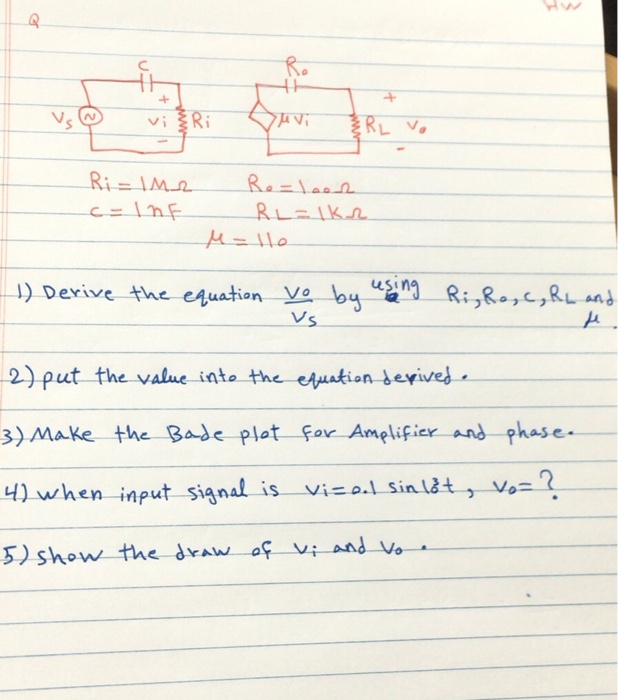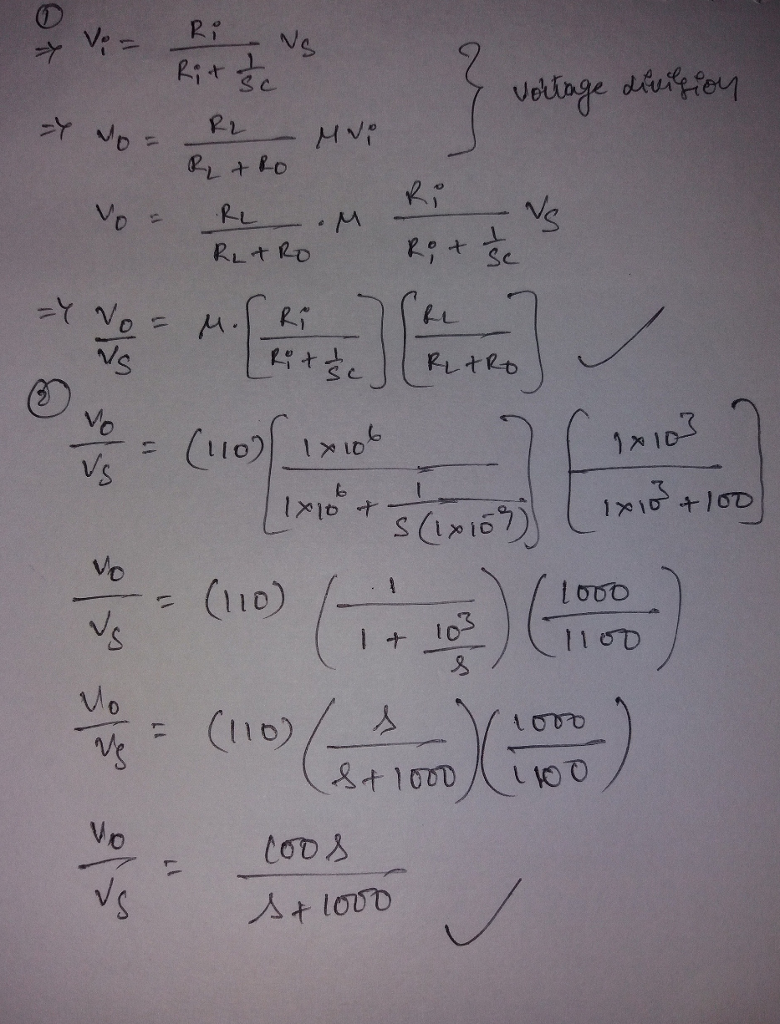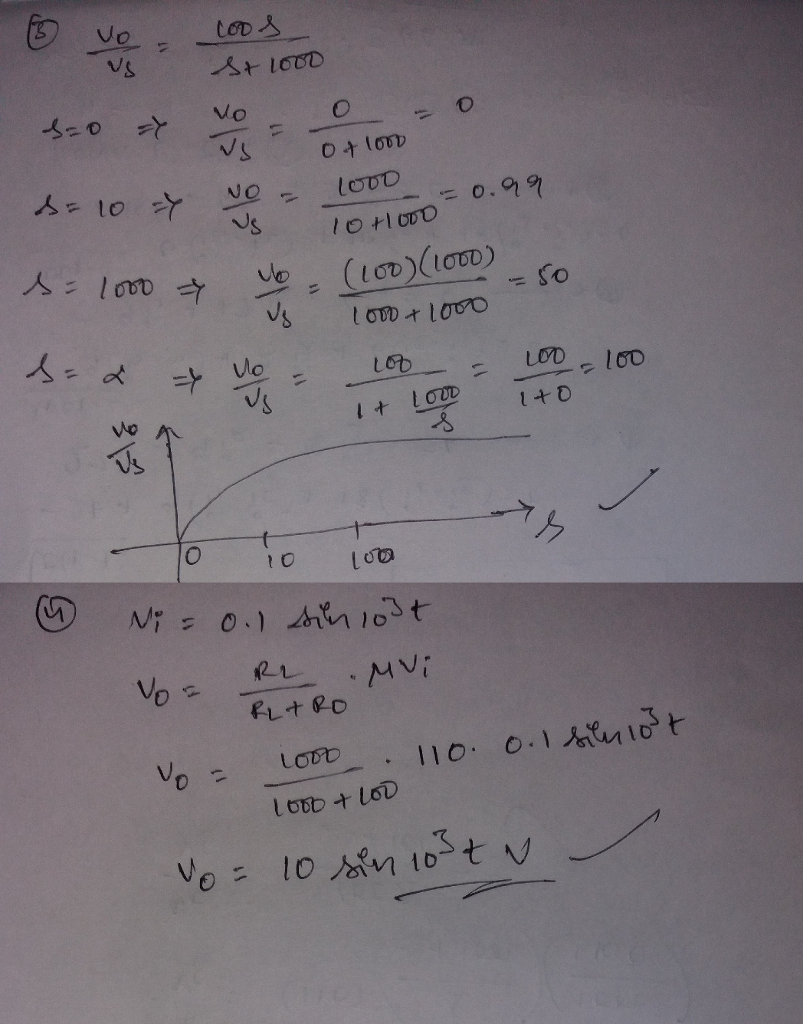# Homework Solution: Please I need solution for this problem…

Please I need solution for this problemDerive the equation V_o/V_s by using R_i, R_o, c, R_L and put-the value into the-equation derived. Make the Bade plot fox Amplifier and phase. when input signal is vi = 0.1 sin 10^3 t, V_o = ? show the draw of V_i and V_o.